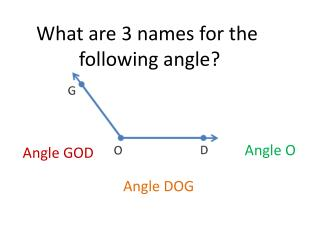DownloadDownload PresentationWhat are 3 names for the following angle?

# What are 3 names for the following angle?

Download Presentation## What are 3 names for the following angle?

- - - - - - - - - - - - - - - - - - - - - - - - - - - E N D - - - - - - - - - - - - - - - - - - - - - - - - - - -
##### Presentation Transcript

1. What are 3 names for the following angle? Angle O Angle GOD Angle DOG

2. A type of polygon has a total of 3 angles. One angle measure 102°. What is the name of the polygon? Obtuse Triangle

3. What is the measure of angle QPC? What is the measure of angle APQ? 50° 150° Q

4. Draw a polygon that has all perpendicular sides.

5. Name some shapes that have MORE than 4 vertices?

6. Mrs. Madison decides to cut the cookie cake below into 6 slices. What would be the angle measure of each slice? 60° (360° ÷ 6 slices) What if she cut the cookie cake into 8 slices. What would the angle measure of each slice be then? 45° (360° ÷ 8 slices)

7. Green 67° Blue Yellow Red The circle above is divided into 4 sections. Each section has a certain angle measure: Red - 113° Green - 132° Blue - 48° What is the angle measure of the yellow section?

8. Aidan draws an angle that measures 100°. He decides to draw a ray that divides his angle equally in half. He thinks he needs a protractor to measure the 2 new angles. Is he right or wrong? What is the measure of the 2 new angles? Aidan does not need a protractor. • He can just divide 100° by 2. Each angle • measures 50°.

9. How many 1° turns are in a circle? 360

10. What fraction of a circle is a 75° turn? 75 360

11. What type of angle is represented by Angle C in the polygon below? Obtuse Angle Acute Angle What about Angle K?

12. Henry drew rectangle EFGH. He drew a line segment that connected vertex E to vertex G. If angle FEG measures 38°, what does angle HEG measure? E F H G 52°

13. If you look at a clock, the hands form angles. When the big hand is on the 12 and the little hand is on the 2, the measure of the angle formed is 60°. What would the measure of the angle be when the big hand is on the 12 and the little hand is on the 8? 240°

14. What fraction of a circle is a 180° turn? 180 360

15. How many lines of symmetry does the following shape have? 5

16. Which streets are parallel to each other? Fillmore, Webster, and Buchanan Streets Jefferson, Beach, and Bay Streets

17. How many lines of symmetry does the following shape have? 2

18. Name some figures that must have both parallel and perpendicular sides. Square Rectangle

19. A type of polygon has a total of 3 angles. Each angle measures under 90°. What is the name of the polygon? Acute Triangle

20. Draw a quadrilateral with four right angles that is not a square.

21. Which streets are perpendicular to each other? Fillmore and Jefferson Webster and Jefferson Fillmore and Beach Webster and Beach Fillmore and Bay Webster and Bay Buchanan and Bay Buchanan and Beach

22. Look at the angle below. If angle REM measures 138°, what is the measure of angle DER? 48° D R E M

23. Y X W V U T Z What is the name of figure XYZU? Trapezoid Right Angle What is the name of figure WVU?